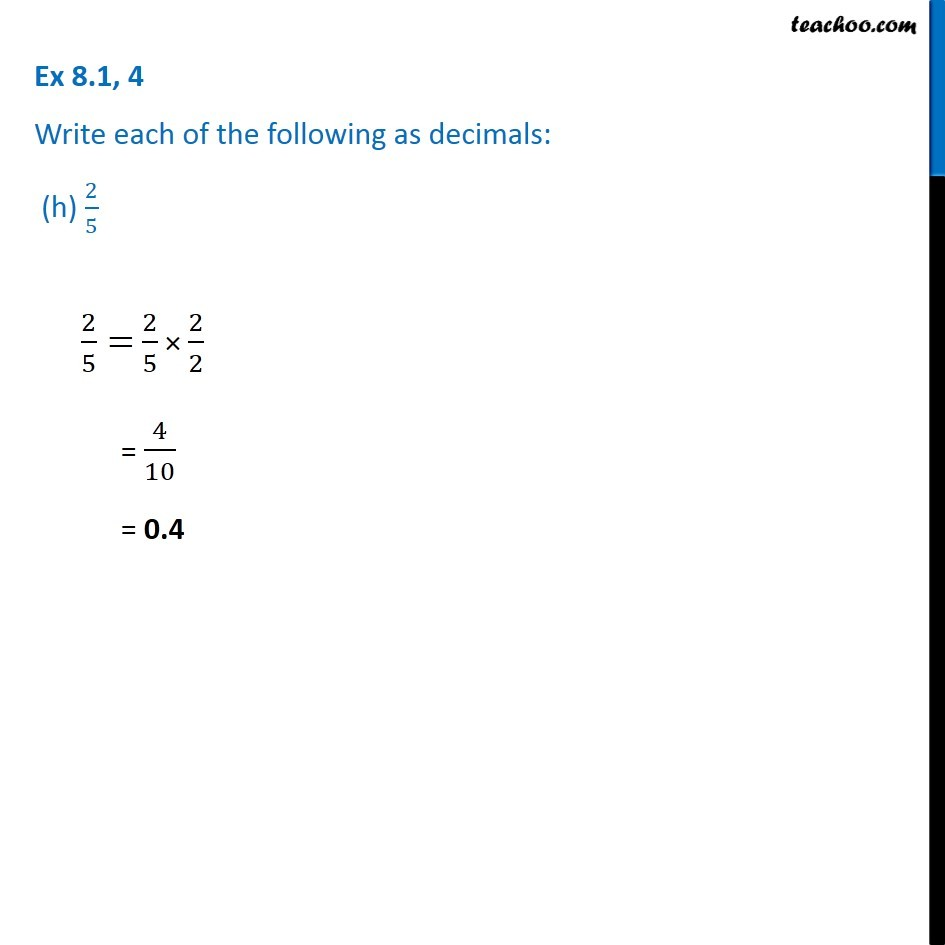1. Chapter 8 Class 6 Decimals
2. Serial order wise
3. Ex 8.1

Transcript

Ex 8.1, 4 Write each of the following as decimals: (h) 2/5 Ex 8.1, 4 Write each of the following as decimals: (h) 2/5 2/5= 2/5 × 2/2 = 4/10 = 0.4